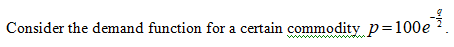# The demand function for a certain commodity is given by p = 100e−q/2. (p is the price per unit and q is the number of units.)(a) At what price per unit will the quantity demanded equal 6 units? (Round your answer to the nearest cent.)\$ (b) If the price is \$1.99 per unit, how many units will be demanded, to the nearest unit? units

Question

The demand function for a certain commodity is given by p = 100eq/2. (p is the price per unit and q is the number of units.)

(a) At what price per unit will the quantity demanded equal 6 units? (Round your answer to the nearest cent.)

(b) If the price is \$1.99 per unit, how many units will be demanded, to the nearest unit?
units
check_circleExpert Solution
Step 1Step 2

(a) The price per unit is evaluated as follows for q = 6.

Step 3

Thus, p = \$4.98.

(b) The price per unit is p = \$1.99. The number of units...

### Want to see the full answer?

See Solution

#### Want to see this answer and more?

Solutions are written by subject experts who are available 24/7. Questions are typically answered within 1 hour*

See Solution
*Response times may vary by subject and question
Tagged in

### Applications of Mathematics